2022: SklogWiki celebrates 15 years on-line

# Martyna-Tuckerman-Tobias-Klein barostatThis article is a 'stub' page, it has no, or next to no, content. It is here at the moment to help form part of the structure of SklogWiki. If you add sufficient material to this article then please remove the {{Stub-general}} template from this page.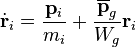$\dot{\mathbf {r}}_i = \frac{{\mathbf {p}}_i}{m_i} + \frac{\overline{\mathbf {p}}_g}{W_g} {\mathbf {r}}_i$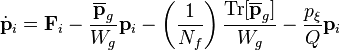$\dot{\mathbf {p}}_i = {\mathbf {F}}_i - \frac{\overline{\mathbf {p}}_g}{W_g} {\mathbf {p}}_i - \left(\frac{1}{N_f}\right) \frac{\mathrm{Tr}[ \overline{\mathbf {p}}_g ]}{W_g} - \frac{p_{\xi}}{Q} {\mathbf {p}}_i$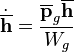$\dot{\overline{\mathbf {h}}} = \frac{\overline{\mathbf {p}}_g {\overline{\mathbf {h}}} }{W_g}$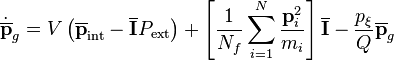$\dot{\overline{\mathbf {p}}}_g = V \left({\overline{\mathbf {p}}}_{\mathrm {int}} - {\overline{\mathbf {I}}} P_{\mathrm {ext}} \right) + \left[ \frac{1}{N_f} \sum_{i=1}^N \frac{{\mathbf {p}}_i^2 }{m_i} \right] {\overline{\mathbf {I}}} - \frac{p_{\xi}}{Q}{\overline{\mathbf {p}}}_g$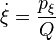$\dot\xi= \frac{p_{\xi}}{Q}$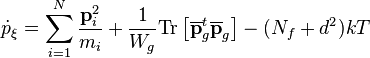$\dot p_{\xi} = \sum_{i=1}^N \frac{{\mathbf {p}}_i^2 }{m_i} + \frac{1}{W_g} \mathrm{Tr}\left[ {\overline{\mathbf {p}}}_g^t {\overline{\mathbf {p}}}_g \right] - (N_f + d^2) kT$